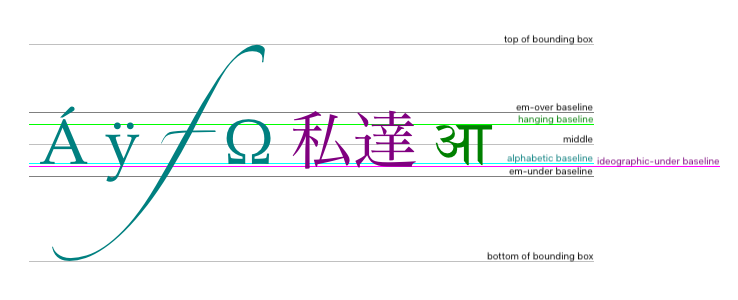Drawing lines along text baselines

I'm not very familiar with the world of TeX and its flavors, and I'm having trouble evaluating whether it fits my needs before I dive into it. TeX and its ilk can draw horizontal line strokes, but can any of them align the lines at the various typographic baselines of running text (hanging, alphabetic, ideographic)—producing something like the image below from the WHATWG HTML5 canvas baseline spec?• I would expect this to be difficult as TeX internally does not know much about each character -- it just sees a bounding box. However, each font contains meta information but I don't know how accessible it is. LuaTeX is probably the best bet here. – Christian Lindig Oct 23 '15 at 16:52
• – Steven B. Segletes Oct 23 '15 at 17:06
• Bounding box, em square, middle and alphabetic baseline are known to all tex flavours. Values for ideographic and hanging baseline exist only in open type fonts and you would need xetex or luatex. The last is imho certainly able to extract the values and to use them, but I don't know if someone has already implemented code for it. I also don't know if xetex can use the values. – Ulrike Fischer Oct 23 '15 at 17:20
• Actually, every character (box) has a height (distance from top to basline) and depth (distance from bottom to baseline). In particular, the height and depth of \strut mark the top and bottom for all characters. One can also express them as fractions of \baselineskip. – John Kormylo Oct 23 '15 at 19:03

Some values are accessible in TeX, e.g.:

\documentclass[12pt]{article}
\usepackage[T1]{fontenc}
\usepackage{lmodern}
\usepackage{color}
\setlength{\fboxsep}{0pt}
\setlength{\fboxrule}{.3pt}
\setcounter{secnumdepth}{0}
\begin{document}

\subsection{Bounding box}
\begin{quote}
\color{red}
\Huge
\def\test#1{\fbox{\color{blue}#1}}
\test{\A} \test{\sffamily C} \test{\itshape f}
\test{$\cdot$} \test{\_}%
\end{quote}

\subsection{Baseline}
\begin{quote}
\Huge
\sbox0{\color{blue}\,abcfgjpq=-\_x\,}
\noindent
\rlap{\color{red}\vrule width\wd0 height.15pt depth.15pt}%
\usebox0
\end{quote}

\subsection{Math axis}
\begin{tabular}{@{}lll@{}}
\textbullet & \verb|\sbox0{$\vcenter{}$}\the\ht0| & 6.22pt\\
\textbullet & \verb|\the\fontdimen22\textfont2\relax| & 6.22pt\\
\end{tabular}
\begin{quote}
% Math axis via an empty \vcenter`, which vertically
% centers around the math axis.
\sbox0{\Huge$\vcenter{}$}% \vcenter centers around the math axis
\sbox2{\Huge\color{blue}\,${=}{+}{\cdot}{-}{\equiv}{\times}$\,}
\noindent
\rlap{\color{red}%
\vrule width\wd2 height\dimexpr\ht0+.15pt\relax
depth-\dimexpr\ht0-.15pt\relax
}%
\usebox2
\end{quote}

\subsection{Corpus size, x-height}
Font dependent unit: \verb|1ex|
\begin{quote}
\sbox0{\Huge\color{blue}\,abcdefghijklmnopqrstuvwxyz\,}
\noindent
\rlap{\color{red}\Huge
\vrule width\wd0 height\dimexpr1ex+.15pt\relax
depth-\dimexpr1ex-.15pt\relax
}%
\usebox0
\end{quote}

\subsection{Em}
Font dependent unit: \verb|1em|
\begin{quote}
$\vcenter{\color{red}\hbox{\Huge\rule{1em}{1em}}}$\quad
1\,em $\times$ 1\,em
\end{quote}
\end{document}Bounding box

TeX is not a rasterizer, thus it does not know the exact bounding box. Instead it relies on the glyph bounding box reported by the font:

• Side bearings are included (left and right white margin).

• Baseline is included (see underscore or middle dot in the example above).

• There are cases, where the glyph bounding box from the font can be larger or smaller than the actual bounding box. Often this is part of the font design, sometimes font bugs.